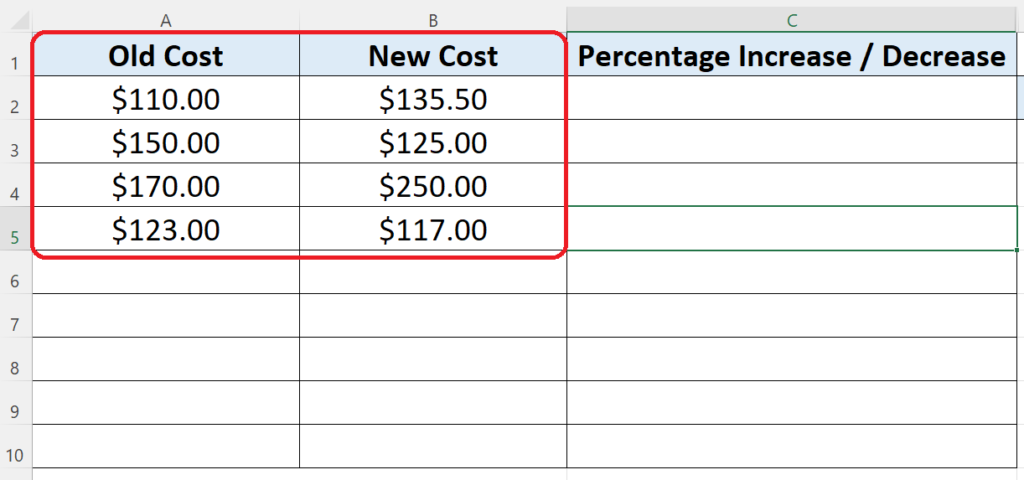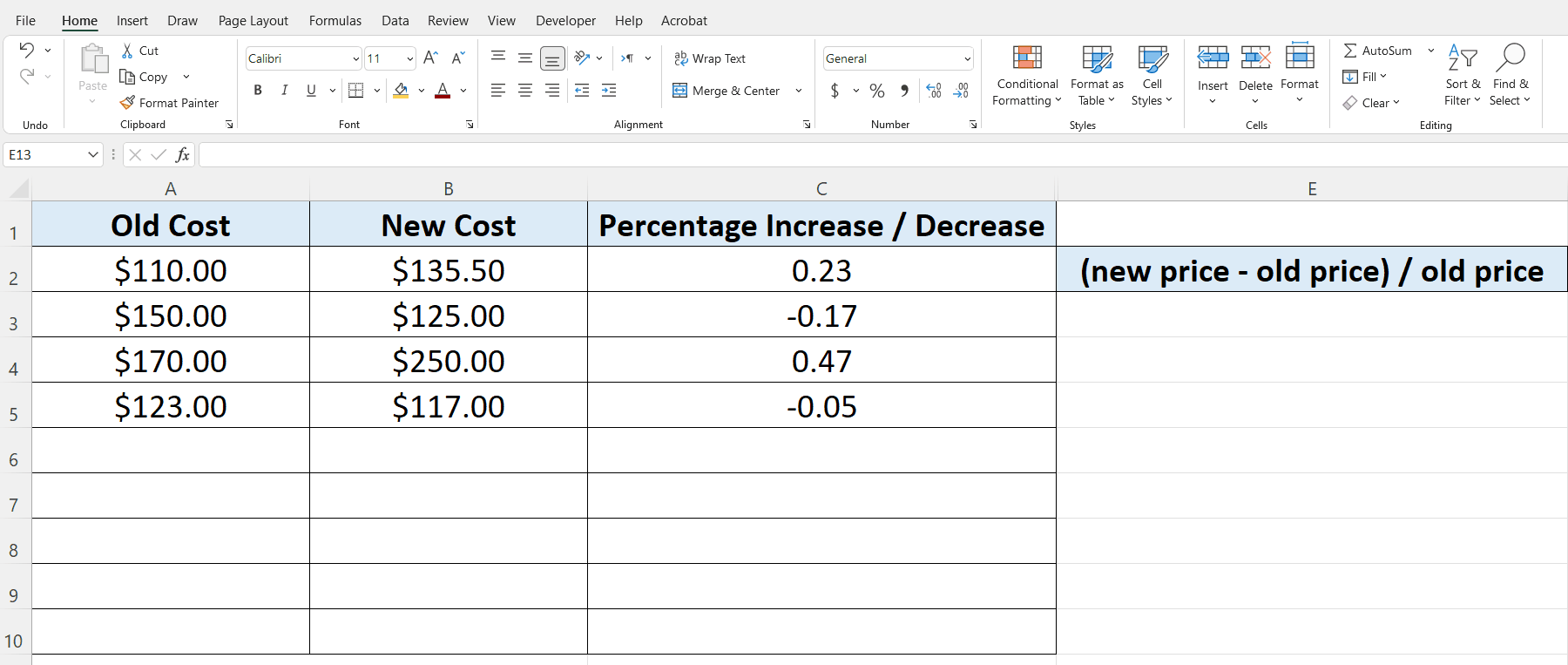# How to calculate percentage difference of two numbers in ExcelPercentage difference can be calculated very easily and quickly using excel. Let’s see this by taking a simple example data set as shown above.

In our data set we wish to find out the percentage increase or decrease in the cost of some items. Standard formula to calculate the percentage difference between two values is as follows;

(new value – old value) / old value

Let’s do this by following these steps;We can use MS Excel to perform basic to complex mathematical calculations. This software provides very handy tools to do these mathematical calculations. One such operation is calculating the percentage difference between two numbers.

### Step 1 – Calculate the Percentage Difference– Choose a suitable cell where you wish to implement the formula. In this case we have chosen C2 to implement this formula.

– Use the following formula in that cell and press enter key.
= (B2 – A2) / A2

That’s how we calculate the percentage difference of two values.

### Step 2 – Show the values in percentage format– By using the formula mentioned in step 1 we calculated the percentage difference between the values however, the result will be shown in numbers by default and not in percentage.

– So to display the percentage difference in percentage actually, first select the values which you want to be displayed as percentage.

– Then at HOME tab go to Number group and press the % button as shown in the figure above.

– The positive percentage shows an increase in the cost while the negative percentage shows a decrease in the value as compared to the original value.